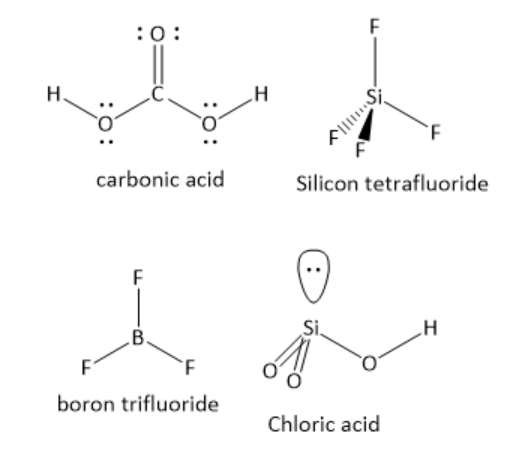Among the following compounds, which compound is polar as well as exhibit $s{p^2}$ hybridization by the central atom?A ) ${{\text{H}}_{\text{2}}}{\text{C}}{{\text{O}}_{\text{3}}}$B ) ${\text{Si}}{{\text{F}}_{\text{4}}}$C ) ${\text{B}}{{\text{F}}_{\text{3}}}$D ) ${\text{HCl}}{{\text{O}}_{\text{3}}}$Verified
114.9k+ views
Hint: First determine the hybridization of the central atom in each compound. For this, determine the number of bonding domains and the number of lone pairs of electrons around central atom. Then determine if the individual bond dipoles cancels each other or not based on the molecular geometry.In ${{\text{H}}_{\text{2}}}{\text{C}}{{\text{O}}_{\text{3}}}$the central carbon atom has one carbon -oxygen double bond and two carbon-oxygen single bonds. The number of lone pair of electrons is zero. The central carbon atom has three bonding domains and zero lone pairs of electrons. Hence, the carbon atom is $s{p^2}$ hybridized. The geometry around the carbon atom is trigonal planar. The individual bond dipoles do not cancel each other. Hence, it is a polar molecule.

In ${\text{Si}}{{\text{F}}_{\text{4}}}$the central silicon atom has four silicon-fluorine single bonds. The number of lone pair of electrons is zero. The central silicon atom has four bonding domains and zero lone pairs of electrons. Hence, the silicon atom is $s{p^3}$ hybridized. The geometry around silicon atom is tetrahedral. The individual bond dipoles cancel each other. Hence, it is a non-polar molecule.

In ${\text{B}}{{\text{F}}_{\text{3}}}$the central boron atom has three boron-fluorine single bonds. The number of lone pair of electrons is zero. The central boron atom has three bonding domains and zero lone pairs of electrons. Hence, the boron atom is $s{p^2}$ hybridized. The geometry around the boron atom is trigonal planar. The individual bond dipoles cancel each other. Hence, it is a non-polar molecule.

In ${\text{HCl}}{{\text{O}}_{\text{3}}}$the central chlorine atom has two chlorine-oxygen double bonds and one chlorine-oxygen single bond. The number of lone pairs of electrons is one. The central chlorine atom has three bonding domains and one lone pair of electrons. Hence, the chlorine atom is $s{p^3}$ hybridised. The geometry around the chlorine atom is tetrahedral. The individual bond dipoles do not cancel each other. Hence, it is a polar molecule.

Hence, the option A ) ${{\text{H}}_{\text{2}}}{\text{C}}{{\text{O}}_{\text{3}}}$ carbonic acid is the correct answer.

Additional information: Steric number five is associated with $s{p^3}d$ hybridization and trigonal bipyramidal shape, when five bond pairs of electrons and zero lone pair of electrons are present. When four bond pairs of electrons and zero lone pair of electrons are present, the shape is see-saw.
Steric number seven is associated with $s{p^3}{d^3}$ hybridization and pentagonal bipyramidal shape

Note:
When n number of atomic orbitals undergo hybridization, n number of degenerate hybrid orbitals are obtained. Some of these hybrid orbitals are occupied by the bond pairs of electrons and the remaining hybrid orbitals are occupied by the lone pair of electrons. By counting the number of bond pairs of electrons and the number of lone pairs of electrons, the number of hybrid orbitals and the type of hybridization can be determined.